### Day 83 - Review - 05.18.15

• Unit 6 Test
• Tuesday, May 19th

Questions

Bell Ringer
1. The variables x and y are inversely related. y = 3 when x = 6. Find y when x = 9

1. 1

2. 2

3. 9

4. 0

5. none of the above

2. Write the following as mixed expression: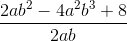1.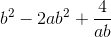2.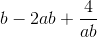3.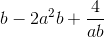4.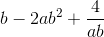5. none of the above

3. Simplify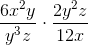.

1.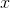2.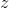3.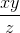4.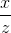5. none of the above

4. Simplify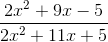.

1. -1

2.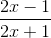3.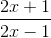5. none of the above

5. Find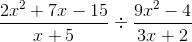.

1.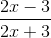2.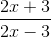3.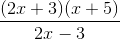4.5. none of the above

Review
• Fractions
• Operations with Fractions (+, -, *, /)
• Simplifying Fractions
• Rationals

Lesson
• Review

Exit Ticket
• Posted on the board!

Essential Question(s)
• Review
Skills
1. Define and give an example of a rational expression.
2. Find the excluded values of a rational expression.
3. Simplify rational expressions.
4. Multiply rational expressions.
5. Divide rational expressions.
6. Divide polynomials using long division.
7. Add/subtract rational expressions with common denominators.
8. Add/subtract rational expressions with uncommon denominators.
9. Simplify complex fractions and mixed numbers.
10. Solve rational equations.
11. Check for extraneous solutions.
12. Apply rational equations to solve real world problems.

#### In-Class Help Requests

Standard(s)
• CC.9-12.A.REI.2 Understand solving equations as a process of reasoning and explain the reasoning. Solve simple rational and radical equations in one variable, and give examples showing how extraneous solutions may arise.
• CC.9-12.A.APR.6 Rewrite rational expressions. Rewrite simple rational expressions in different forms; write a(x)/b(x) in the form q(x) + r(x)/b(x), where a(x), b(x), q(x), and r(x) are polynomials with the degree of r(x) less than the degree of b(x), using inspection, long division, or, for the more complicated examples, a computer algebra system.
• CC.9-12.A.REI.11 Represent and solve equations and inequalities graphically. Explain why the x-coordinates of the points where the graphs of the equations y = f(x) and y = g(x) intersect are the solutions of the equation f(x) = g(x); find the solutions approximately, e.g., using technology to graph the functions, make tables of values, or find successive approximations. Include cases where f(x) and/or g(x) are linear, polynomial, rational, absolute value, exponential, and logarithmic functions.*
• CC.9-12.A.CED.1 Create equations that describe numbers or relationship. Create equations and inequalities in one variable and use them to solve problems. Include equations arising from linear and quadratic functions, and simple rational and exponential functions.*

Mathematical Practice(s)
• #1 - Make sense of problems and persevere in solving them
• #2 - Reason abstractly and quantitatively
• #7 - Look for and make use of structure